• 2
•
• 1
•
•
•
•
•
3
Shares

# CAPACITOR AND CAPACITANCE

In this article we are going to talk about Capacitor and capacitance, definition, formula, types of capacitor and its uses in detail so stay tuned with us till end.

## CAPACITOR?

A capacitor is a two terminal electrostatic device which has the ability to store electric energy in the form of electric charge. It is commonly known as condenser. It consist of two conducting plates which is seperated at some distance.

This distance (space between the two conducting plates) is filled up by the vaccum or non conducting material knows as dielectric.It stores the electrical energy by holding opposite charges on its terminal which is apart by the small distance. The basic design of capacitor is parallel plate capacitor whose metal plates is seperated at some distance.

Note that a capacitor as whole is electrically neutral.

## CAPACITANCE

We know that capacitor store electric energy by holding electrical charges. But every capacitor has different capacity of holding electrical charges. The ability of capacitors of holding electrical charges is called capacitance

### FINDING THE VALUE OF CAPACITANCE

Let conducting plates having the charge +q and -q. (If charge on the first plate is either positive or negative then the other plate will have the charge opposite to the charge on the first plate)

We know that the electric field between the plate is directly proportional to the charges on the plate. If higher the charge on the plate then the higher the electric field between the plates.

And we also know that electric field is directly proportional to the potential difference (V). Hence from this we can say that electric charge is directly proportional to the potential difference.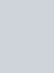This constant of proportionality is called the capacitance of the capacitor.

Capacitance is the ratio of  change of the electric charge of a system to the corresponding change of the potential difference.

The capacitance C depends on the value of electric charge and potential difference as well as shape, size, dielectric and seperation distance between the plates of a capacitor.

The SI unit of capacitance is farad. It dimensional formula is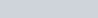.

The definition of one farad of capacitance – if a capacitor hold one coulomb of electric charge at per unit potential difference then the capacitor has the capacitance of one farad. Actually farad is a very big unit, the most common units are its sub multiples.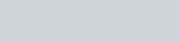The capacitance of any capacitor can be either fixed or variable depending on their usage.

### FACTORS AFFECTING CAPACITANCE

Capacitance of a capacitor is affected by various factors but some of the main factors are –

1. DIELECTRIC CONSTANT

Capacitance of the capacitor is directly proportional to the dielectric constant of the insulating material. If dielectric constant K increases then the capacitance of the capacitor also increases.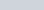2. SEPERATION DISTANCE BETWEEN THE PLATES

Capacitance is inversely proportional to the seperation distance between the plates. Smaller the distance larger the capacitance.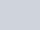3. SURFACE AREA OF THE PLATES

Capacitance of the capacitor is directly proportional to the surface area of the capacitor. If surface area of the plates is large then the it can hold large amount of electric charge. And capacitance is directly proportional to the electric charge, larger the charge larger the capacitance.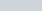4. SIZE OF THE CAPACITOR

If the size of the capacitor is large then the potential difference at the terminal of the plates will be smaller. And the smaller the potential difference then the larger the capacitance because capacitance is inversely proportional to the potential difference.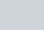## WORKING OF A CAPACITOR

Now let’s talk how a capacitor works?

Let’s consider the most basic structure of a capacitor i.e the parallel plate capacitor. This capacitor consist of two metal plates which is seperated by some distance. And the space between the plates is filled with the dielectric material.

When we connect this capacitor to a DC voltage source. The positive terminal of the voltage source is connected to the end of plate 1 and the negative terminal to the end of plate 2. (You can connect positive terminal to any of the plate, it is your choice. NO ISSUE).

When the potential of the battery is applied across the capacitor then the plate 1 become positive with respect to the plate 2. In steady state condition electric current wants to flow from the capacitor but it can’t flow through them because both plates is seperated by the insulating material.

The plate 1 accumulates positive charges from the battery and the plate 2 accumulates negative charges of the same quantity from the battery. Due to the accumulation of electric charges a electric field generated across the capacitor.

After a point, capacitor holds maximum amount of electric charge as per it’s capacitance with respect to the voltage supplied. The time taken by the capacitor to holds the maximum amount of charge is called the charging time of the capacitor.

But if we remove the power supply then the both plates of the capacitor hold the electric charges for sometimes and behaves as a electrical source. But it starts loosing it’s stored charge gradually, if we connect it to any load. The time taken by the capacitor to looses it’s stored charge completely is called the discharging time of the capacitor.

1. What is capacitors and capacitance?

Ans – Capacitor is a electrostatic device which stores electrical energy in the form of electric charges. And the capacitance is the ability of the Capacitor to hold electric charges.

2. What is the working principle of the capacitor?

Ans –  A capacitor works on the principle that the capacitance of a conductor increases appreciably when an earthed conductor is brought near it.

3. Why is a capacitor called a condenser?

Ans – Condenser are those devices which condense something, here Capacitor is called condenser because Capacitor condense electric charges on its plates surface as per it’s capacitance.

4. Is a capacitor a battery?

Ans – you can say but there are some key differences between Capacitor and battery. Capacitor stores electrical energy in an electric field but a battery stores electric energy in chemical form.

5. What is the net charge on the Capacitor?

Ans– It is true that Capacitor stores electrical energy in the form of electric charges. It consists of two metal plates which is seperated by the some distance having equal and opposite charges. Having equal and opposite charges on the plates this makes the Capacitor electrically neutral. So a Capacitor is electrically neutral.